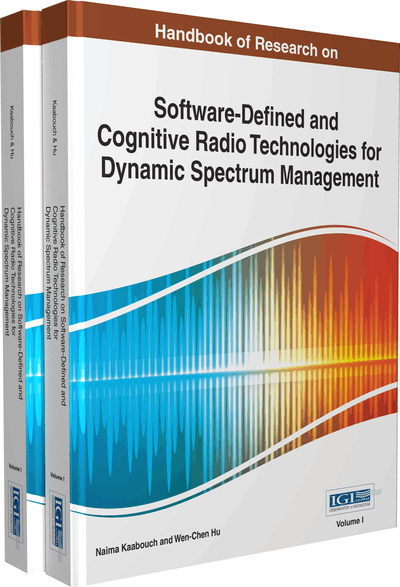# Modeling and Performance Evaluation

Yuehong Gao, Zhidu Li, Guoting Zhang, He Bai
DOI: 10.4018/978-1-4666-6571-2.ch028
OnDemand:
(Individual Chapters)
Available
\$29.50
No Current Special Offers

## Abstract

Performance evaluation and analysis are of key importance to obtain deep understanding of cognitive radio networks. Some effects have been made to model and analyze the performance of cognitive radio networks. In the literature, there are two methodologies: queuing theory/Markov chain-based analysis and stochastic network calculus-based analysis. These two methodologies rely on different mathematical basics and modeling approaches. Thus, they lead to different output metrics on various viewpoints. This chapter aims to give an overall introduction to both methodologies. First, the fundamental models used in queuing/Markov chain-based analysis are presented, followed by their applications in cognitive radio networks. Then, network calculus basics are introduced with the modeling and application in performance analysis of the cognitive radio network.
Chapter Preview
Top

## Queuing Theory/Markov Chain Based Analysis

In this section, queuing theory/Markov chain basics and the priority mechanism in cognitive radio networks will be introduced. Then, cognitive radio networks will be modeled and some application instances will be presented and discussed.

## Key Terms in this Chapter

Delay: The time period between the arrival time of a request/packet/bits and the time when it is completely served.

Queuing Theory: A mathematical method used to analyze the performance of a network in which users are queued and served by a certain policy.

Markov Chain: A mathematical system containing at least two states and the system's state transits from one state to another memorylessly, i.e. the next state only depends on the current state and is irrelevant to the previous states.

Priority System: A system that serves different users/processes in a particular order based on their priority levels, where high priority means more chances and privileges.

Delay-Constrained Capacity: A maximum supported arrival rate of the input flow which can satisfy a given delay constraint.

Network Calculus: A newly developed queuing problem modeling tool for service guarantee analysis, which can be used to deal with flow problems in networks.

Performance Evaluation: Using proper models, both theoretically and simulatively, to estimate a system’s performances from different aspects, such as waiting time, queue length, packet loss probability, capacity and etc.

Cognitive Radio Network: An intelligent wireless network which can improve the wireless spectrum utilization by allowing unlicensed users to detect and transmit through spectrum holes.

Backlog/Queue Length: The length of requests/packets/bits that are waiting in a queue for service.

## Complete Chapter List

Search this Book:
Reset